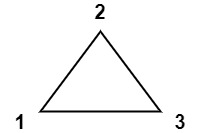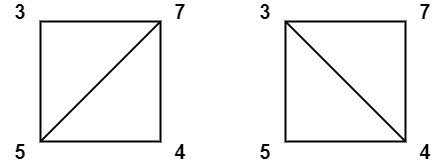1039. Minimum Score Triangulation of Polygon
Medium
1.5K
146

You have a convex `n`-sided polygon where each vertex has an integer value. You are given an integer array `values` where `values[i]` is the value of the `ith` vertex (i.e., clockwise order).

You will triangulate the polygon into `n - 2` triangles. For each triangle, the value of that triangle is the product of the values of its vertices, and the total score of the triangulation is the sum of these values over all `n - 2` triangles in the triangulation.

Return the smallest possible total score that you can achieve with some triangulation of the polygon.

Example 1:```Input: values = [1,2,3]
Output: 6
Explanation: The polygon is already triangulated, and the score of the only triangle is 6.
```

Example 2:```Input: values = [3,7,4,5]
Output: 144
Explanation: There are two triangulations, with possible scores: 3*7*5 + 4*5*7 = 245, or 3*4*5 + 3*4*7 = 144.
The minimum score is 144.
```

Example 3:```Input: values = [1,3,1,4,1,5]
Output: 13
Explanation: The minimum score triangulation has score 1*1*3 + 1*1*4 + 1*1*5 + 1*1*1 = 13.
```

Constraints:

• `n == values.length`
• `3 <= n <= 50`
• `1 <= values[i] <= 100`
Accepted
35K
Submissions
62.6K
Acceptance Rate
55.9%

Seen this question in a real interview before?
1/4
Yes
No

Discussion (0)

Related Topics